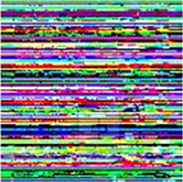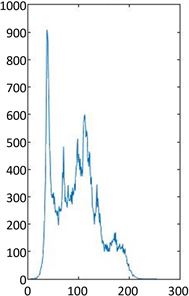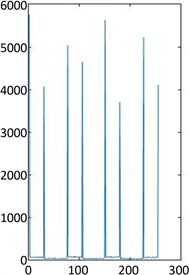﻿ 基于线性变换的彩色数字图像置乱算法

基于线性变换的彩色数字图像置乱算法Color Digital Image Scrambling Algorithm Based on Linear Transformation

Abstract: There are some problems that traditional color digital image scrambling algorithm has: small key space, weak anti-attack and low encryption intensity. In this paper, we present a new algorithm of color digital image scrambling algorithm based on linear transformation. In order to achieve the purpose of pixel scrambling, it randomly generates three matrices, operates with the three RGB matrices of the original color digital image, and then it will disrupt the position of the pixel scrambling. The simulation results show that the algorithm can change the gray image features of image, and has the advantages of stability, strong randomness and strong anti-attack.

1. 引言

2. 基于线性变换的图像置乱算法

$R={\left({r}_{ij}\right)}_{k×r},\text{}\left(i=1,2,\cdots ,k,\text{\hspace{0.17em}}j=1,2,\cdots ,r\right)$

$G={\left({g}_{ij}\right)}_{k×r},\text{}\left(i=1,2,\cdots ,k,\text{\hspace{0.17em}}j=1,2,\cdots ,r\right)$

$B={\left({b}_{ij}\right)}_{k×r},\text{}\left(i=1,2,\cdots ,k,\text{\hspace{0.17em}}j=1,2,\cdots ,r\right)$

${R}^{\prime }={P}_{1}R$ (1)

${G}^{\prime }={P}_{2}G$ (2)

${B}^{\prime }={P}_{3}B$ (3)

$R={P}_{1}^{-1}{R}^{\prime }$ (4)

$G={P}_{2}^{-1}{G}^{\prime }$ (5)

$B={P}_{3}^{-1}{B}^{\prime }$ (6)

3. 仿真实验与分析原图像加密后解密后

Figure 1. Lena image scrambling and reduction map灰度图灰度直方图

Figure 2. The gray and grayscale histograms of the original image灰度图灰度直方图

Figure 3. The grayscale and histogram of the encrypted image

4. 结语

 董虎胜, 陆萍, 钟宝江. 基于Hénnon映射与模仿变换的图像加密算法[J]. 计算机应用与软件, 2014, 31(5): 291- 294.

 王冬梅, 黄琳, 王金荣. 幻方变换加密数字全息图[J]. 浙江工业大学学报, 2007, 35(1): 116-118.

 文昌辞, 王沁, 刘向宏. 基于放射和复合混沌的图像加密新算法[J]. 计算机研究与发展, 2013, 50(2): 319-324.

 龚黎华, 曾绍阳, 周南润. 基于频谱切割和二维Arnold变换的彩色图像加密算法[J]. 计算机应用, 2012, 32(9): 2599-2602.

 袁玲, 康宝生. 基于Logistic混沌序列和位交换的图像置乱算法[J]. 计算机应用, 2009, 29(10): 2681-2683.

 段雪峰, 关健, 丁勇, 刘云波. 基于多组混沌序列的彩色数字图像置乱算法[J]. 计算机工程,2012, 38(9): 114-120.

 汪太月, 戴燕青. 数字图像置乱算法的研究与比较[J]. 湖北理工学院学报, 2017, 33(4): 425-430.

 郭婷婷, 娄岩, 刘佳, 王艳华. 基于Rossler变换的图像置乱算法[J]. 辽宁师范大学学报, 2017, 40(1): 41-46.

 江帆, 吴小天, 孙伟. 基于稀疏矩阵的Arnold数字图像加密算法[J]. 计算机应用, 2015, 35(3): 726-731.

Top